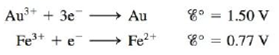Chapter 18, Problem 146CP

Chapter
Section
Textbook Problem

# Consider a cell based on the following half-reactions:a. Draw this cell under standard conditions, labeling the anode, the cathode, the direction of electron flow, and the concentrations, as appropriate.b. When enough NaCl(s) is added to the compartment containing gold to make the [Cl−] = 0.10 M, the cell potential is observed to be 0.31 V. Assume that Au3+ is  reduced and assume that the reaction in the compartment containing gold is Au 3 + ( a q ) + 4 Cl − ( a q ) ⇌ AuCl 4 − ( a q ) Calculate the value of K for this reaction at 25°C.

(a)

Interpretation Introduction

Interpretation:

The reactions taking place in a galvanic cell are given. The sketch of the given galvanic cell and the value of K for the given equilibrium reaction is to be calculated.

Concept introduction:

A galvanic cell is a device that converts chemical energy into electrical energy and therefore spontaneous reaction takes place in the galvanic cell. It consists of two half cells having one electrode each that is present in contact with the solution of its electrolyte.

To determine: The sketch of given galvanic cell with labeled cathode, anode, concentration of ions and direction of electron flow.

Explanation

The reaction taking place at the cathode is,

Au3++3eAuE°red=1.50V

The reaction taking place at the anode is,

Fe2+Fe3++eE°ox=0.77V

Multiply oxidation half-reaction with a coefficient of 3 and then add both the reduction and oxidation half-reactions as,

Au3++3eAu3Fe2+3Fe3++3e

The final equation is,

Au3++3Fe2+Au+3Fe3+

The value of E°cell is given as,

E°cell=E°ox+E°red

Where,

• E°ox is the oxidation potential of the electrode

(b)

Interpretation Introduction

Interpretation:

The reactions taking place in a galvanic cell are given. The sketch of the given galvanic cell and the value of K for the given equilibrium reaction is to be calculated.

Concept introduction:

A galvanic cell is a device that converts chemical energy into electrical energy and therefore spontaneous reaction takes place in the galvanic cell. It consists of two half cells having one electrode each that is present in contact with the solution of its electrolyte.

To determine: The value of K for the given reaction.

The value of equilibrium constant K is 5.24×1015_ .

### Still sussing out bartleby?

Check out a sample textbook solution.

See a sample solution

#### The Solution to Your Study Problems

Bartleby provides explanations to thousands of textbook problems written by our experts, many with advanced degrees!

Get Started